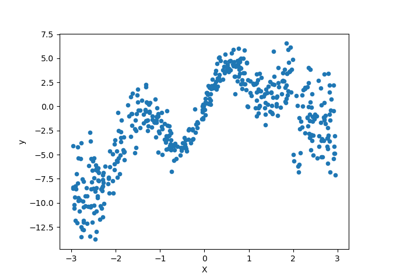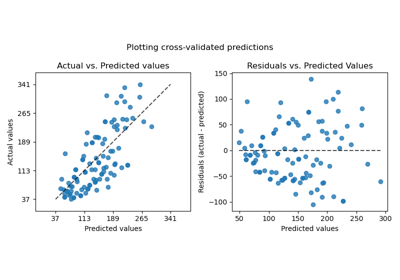# sklearn.model_selection.cross_val_predict¶

sklearn.model_selection.cross_val_predict(estimator, X, y=None, *, groups=None, cv=None, n_jobs=None, verbose=0, fit_params=None, pre_dispatch='2*n_jobs', method='predict')[source]

Generate cross-validated estimates for each input data point

The data is split according to the cv parameter. Each sample belongs to exactly one test set, and its prediction is computed with an estimator fitted on the corresponding training set.

Passing these predictions into an evaluation metric may not be a valid way to measure generalization performance. Results can differ from cross_validate and cross_val_score unless all tests sets have equal size and the metric decomposes over samples.

Read more in the User Guide.

Parameters
estimatorestimator object implementing ‘fit’ and ‘predict’

The object to use to fit the data.

Xarray-like of shape (n_samples, n_features)

The data to fit. Can be, for example a list, or an array at least 2d.

yarray-like of shape (n_samples,) or (n_samples, n_outputs), default=None

The target variable to try to predict in the case of supervised learning.

groupsarray-like of shape (n_samples,), default=None

Group labels for the samples used while splitting the dataset into train/test set. Only used in conjunction with a “Group” cv instance (e.g., GroupKFold).

cvint, cross-validation generator or an iterable, default=None

Determines the cross-validation splitting strategy. Possible inputs for cv are:

• None, to use the default 5-fold cross validation,

• int, to specify the number of folds in a (Stratified)KFold,

• An iterable yielding (train, test) splits as arrays of indices.

For int/None inputs, if the estimator is a classifier and y is either binary or multiclass, StratifiedKFold is used. In all other cases, KFold is used.

Refer User Guide for the various cross-validation strategies that can be used here.

Changed in version 0.22: cv default value if None changed from 3-fold to 5-fold.

n_jobsint, default=None

Number of jobs to run in parallel. Training the estimator and predicting are parallelized over the cross-validation splits. None means 1 unless in a joblib.parallel_backend context. -1 means using all processors. See Glossary for more details.

verboseint, default=0

The verbosity level.

fit_paramsdict, defualt=None

Parameters to pass to the fit method of the estimator.

pre_dispatchint or str, default=’2*n_jobs’

Controls the number of jobs that get dispatched during parallel execution. Reducing this number can be useful to avoid an explosion of memory consumption when more jobs get dispatched than CPUs can process. This parameter can be:

• None, in which case all the jobs are immediately created and spawned. Use this for lightweight and fast-running jobs, to avoid delays due to on-demand spawning of the jobs

• An int, giving the exact number of total jobs that are spawned

• A str, giving an expression as a function of n_jobs, as in ‘2*n_jobs’

method{‘predict’, ‘predict_proba’, ‘predict_log_proba’, ‘decision_function’}, default=’predict’

The method to be invoked by estimator.

Returns
predictionsndarray

This is the result of calling method. Shape:

• When method is ‘predict’ and in special case where method is ‘decision_function’ and the target is binary: (n_samples,)

• When method is one of {‘predict_proba’, ‘predict_log_proba’, ‘decision_function’} (unless special case above): (n_samples, n_classes)

• If estimator is multioutput, an extra dimension ‘n_outputs’ is added to the end of each shape above.

cross_val_score

Calculate score for each CV split.

cross_validate

Calculate one or more scores and timings for each CV split.

Notes

In the case that one or more classes are absent in a training portion, a default score needs to be assigned to all instances for that class if method produces columns per class, as in {‘decision_function’, ‘predict_proba’, ‘predict_log_proba’}. For predict_proba this value is 0. In order to ensure finite output, we approximate negative infinity by the minimum finite float value for the dtype in other cases.

Examples

>>> from sklearn import datasets, linear_model
>>> from sklearn.model_selection import cross_val_predict

## Examples using sklearn.model_selection.cross_val_predict¶